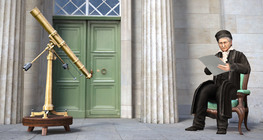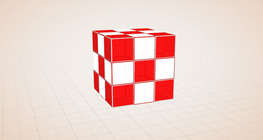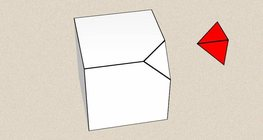# Dice

### Dice

Regular dice can be used for solving statistical and probability exercises.

Mathematics

Keywords

dice, probability, mathematics, experiment in probability

### Related items#### Colouring a cube

Colouring the vertices, edges and faces of a given cube according to the criteria specified in the exercise.#### Cube

This animation demonstrates the components (vertices, edges, diagonals and faces) of the cube, one of the Platonic solids.#### Cuboid

A cuboid is a polyhedron with six rectangular faces.#### Grouping of cuboids

This animation demonstrates various types of cuboids through everyday objects.#### Nets of a cuboid (exercises)

This animation demonstrates the different nets of a cuboid and includes a game.#### The work of Carl Friedrich Gauss

Carl Friedrich Gauss was one of the greatest mathematicians of all time.#### Net of a cube (exercises)

Not all nets consisting of 6 congruent squares are foldable into cubes.#### Cube (exercises)

Edges, diagonals and faces of a cube can be identified by its vertices.#### Cube of cubes

An exercise about the regular hexahedron built from unit cubes to help deepen your knowledge of cubes.#### Cube puzzle

Building cubes shown in several views from the available unit cubes aids spatial vision and other skills.#### Cube sections (exercise)

Examining solids formed by the intersection of a cube and a plane.